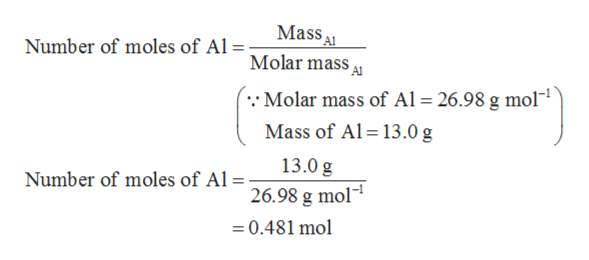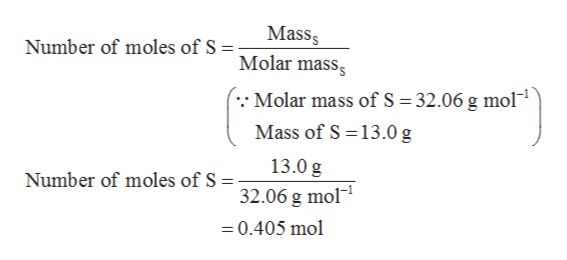# 5. If 13.0grams of aluminum are reacted with 13.0grams of sulfur:a. What is the limiting reagent?b. How many grams of aluminum sulfide are produced?

Question
166 views

5. If 13.0grams of aluminum are reacted with 13.0grams of sulfur:
a. What is the limiting reagent?
b. How many grams of aluminum sulfide are produced?

check_circle

Step 1

Given data:

Step 2

Part (a)

The number of moles of aluminum is calculated as shown below.help_outlineImage TranscriptioncloseMass Molar mass Number of moles of Al =; 26.98 g mol Molar mass of Al Mass of Al 13.0 g 13.0 g Number of moles of Al = 26.98 g mol 0.481 mol fullscreen
Step 3

The number of moles of sulfur is ...help_outlineImage TranscriptioncloseMass Molar masss Number of moles of S ; . Molar mass of S 32.06 g mol Mass of S 13.0 g 13.0 g Number of moles of S - 32.06 g mol 0.405 mol fullscreen

### Want to see the full answer?

See Solution

#### Want to see this answer and more?

Solutions are written by subject experts who are available 24/7. Questions are typically answered within 1 hour.*

See Solution
*Response times may vary by subject and question.
Tagged in

### Chemistry